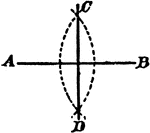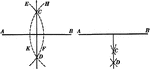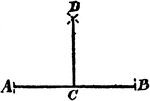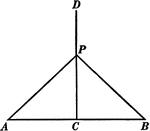### Perpendicular Bisector of a Line

Illustration showing that the perpendicular bisector of a given line is the locus of points equidistant…### Construction Of A Line Divided In Equal Parts

An illustration showing the construction used to divide a line AB into two equal parts; and to erect…### Construction Of A Perpendicular Bisector Of A Straight Line

Illustration of the construction used to make a perpendicular bisector of a straight line.### Construction Of A Perpendicular

An illustration showing the construction used to erect a perpendicular. "With C as a center, draw the…### Triangle With Perpendicular Bisector

Illustration showing a perpendicular bisector of a triangle extended outside of the triangle.